## 单纯形法

单纯形法是针对求解线性规划问题的一个算法，这个名称里的'单纯形'是代数拓扑里的一个概念，可以简单将'单纯形'理解为一个凸集，标准的线性规划问题可以表示为:

min（or max）         f(x)=cx

s.t.            Ax=b

x>=0,b>=0

min     -4x1-x2

s.t.      -x1+2x2<=4

2x1+3x2<=12

x1-x2<=3

x1,x2>=0

1.1 凸集概念

单纯形可以理解为一个凸集，介绍单纯形法之前有必要先来了解一下凸集概念及其性质，在很多机器学习算法中都会穿插凸集的概念，凸集上求最优解是凸优化的一个分支，凸集对于简化问题是有重要意义的。凸集可以用图形表示为一个没有洞的联通区域，如下图所示：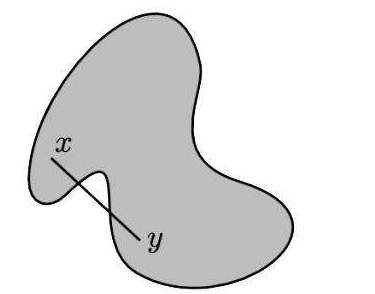Ax=b,Ay=b

x和y之间任意一个元素可以表示为 v= αx+(1-α)y,计算Av=A[αx+(1-α)y]=αb+b-αb=b,显然有v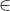S，所以说线性规划中的约束条件描述的是一个凸集。

1.2 凸集的极点（顶点）

从凸集代数定义公式可以发现，凸集中任何一个点都可以用另外两个点线性组合而成，凸集中有一些特殊点用公式表达:x= αx1+(1-α)xx1 ,x2S,如果有且只有x=x1=x2,则称x为极点，或者形象的称为凸集的顶点；从图像上来看顶点不在凸集两个不同点连线上，如下图所示：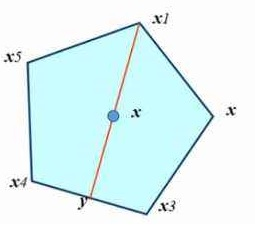对于凸集还有其他一些概念，虽然在单纯形法中没有用到，这里可以顺带提一下，凸集的区域范围可以有界或者是无界的，当凸集有界时，线性规划目标函数是把两个Rn维向量做内积运算得到一个实数R,所以这里本质上是一个有界线性泛函过程，如果没有约束条件的话，显然x向量与c向量呈180度相反方向时可得到最小值，c在这里可以理解为目标函数的梯度。

当凸集无界时时，需要引入方向的概念,设x是Rn维向量，x沿着一个方向延伸得到新的一个点v：v=x+αd    xS;α>0;d>0，如果新的点vS,则称d为可行方向。从线性代数角度来看，方向作为一个向量，可以由其他的方向线性合成，如果凸集一个方向不能由其他方向合成则称这个方向为极方向，这句话也可以反过来理解，凸集的所有可行方向都可以由极方向线性表示，极方向与极点有着对应关系。凸集的可行方向对于理解'可行区域求最优解'、'KKT条件'等非线性规划有着重要的意义。

2.1 可行解、最优解基本可行解

根据上面的描述，凸集中每个元素都满足约束条件，凸集中每个元素称为目标函数的可行解，显然可行解的数量是非常多的，可行解带入目标函数后一般得不到函数的最小值或最大值；如果可行解带入目标函数后可得到最值（最大或最小值），那么这个可行解就成为最优解。接下来介绍基本可行解，基本可行解是实现单纯形法的关键，还是以上面的问题为例，我们新增三变量x3,x4,x5使得约束条件变为等式标准型：

min         -4x1-x2+0x3+0x4+0x5

s.t.          -x1+2x2+x3+0x4+0x5=4

2x1+3x+0x3+x4+0x5=12

x1-x2 +0x3+0x4+x5=3

x1,x2,x3,x4,x5>=0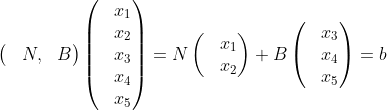从线性空间的角度来理解线性规划问题，目标函数在新增松弛变量后xR5，即目标函数中的x是一个来自5维空间向量，Ax=b的含义是通过线性映射将一个5维空间的向量映射为一个固定的三维向量 b,当然从函数角度来看这个映射过程是多对一的关系，即有多个5维的向量线性映射后变为一个3维向量b,这些5维向量组成的集合之前已经介绍过，是一个凸集，目标函数的含义此时可以表述为，在5维空间组成的凸集中找到一个或多个向量，与一个固定向量c的内积最小。

2.2 基本可行解、凸集顶点（极点）

基本可行解与凸集顶点是等价的，而凸集的顶点在线性规划问题中与最优解又有着密切的联系，首先来证明一个重要的定理：凸集中元素x是基本可行解的充分必要条件为x是凸集的顶点这个定理是实现单纯型法的关键，下面来详细证明一下：

先来证明这个定理必要性:即基本可行解一定是凸集的顶点，设x是基本可行解，根据之前分析可以知道向量x中必有前k个分量大于0，其余分量等于0，这个k个分量对应的是矩阵k个线性无关的列（基），设可行区S内有x1,x2,x1≠x2,根据凸集的定义设基本可行解x=αx1+(1-α)x2,由于基本可行解x前k个分量大于0而其余的分量等于0，又由于α选择的任意性，所以当i>=k+1时，必有x1[i]=x2[i]=0 (x1[i]代表x1第i个分量)。又因为Ax1=Ax2=b，即A(x1-x2)=0,已经知道x1,x2的分量从k+1开始都是0，此时就可以得到，这里Ai是矩阵中几个线性不相关的列，前面已经知道x1≠x2，则Ai的系数x1[i]-x2[i]必然有不等于0的情形，而如果出现不等于0情况说明这些列Ai是线性相关的，这显然与假设矛盾，所以此时可以得到一个结论，没有两个不同的点x1,x2使得有基本可行解x=αx1+(1-α)x2存在要使等式成立必须x=x1=x2,满足这个条件时x显然是凸集顶点（极点）。

接下来证明充分性：x是凸集的顶点则x一定是基本可行解。设顶点x有k个分量大于0，其余等于0，由于约束条件要求x分量必须大于等于0，这样分类显然是成立的。 如果这个k个分量对应矩阵的列是线性无关的，那么顶点x就是基本可行解，这里我们可以用反证法，先假设k个分量对应矩阵列是线性相关的，即存在一个分量不全为0的向量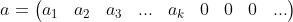使得有等式：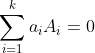，对于顶点x而言，前k分量也有相应的等式: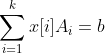,联合这两个等式同时引入一个实数b，b>0,得到下面两组等式：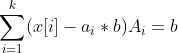2.3 凸集顶点与最优解

线性规划的目标函数是一个线性函数f(x)=cx,这个函数如果没有约束条件的束缚，其值域是正负无穷的，线性函数在定义域上等值线是相互之间平行的向量，等值线不会像非线性规划那样范围逐渐变小，进而变成一个点，这也导致了线性规划问题最值有可能是多个，加入约束条件后，目标函数最值只能在一个凸集中选择，线性规划的等值线运动过程如下图所示：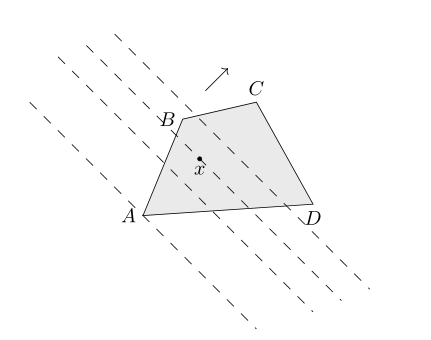我们可以得到这样一个结论，线性规划问题的最值一定在凸集的顶点处。通过之前的分析已经知道，凸集的顶点是满足约束条件的基本可行解，单纯形法的核心思想可以归纳为：找到每一个基本可行解，代入目标函数后计算函数值取其最大或最小值即可。单纯形法上从代数角度是寻找约束条件的每一个基本可行解，从几何意义上来说是遍历凸集的每一个顶点，根据算法的特性有时也称为转轴法。

3.1 入基、出基

单纯形法过程就是不断找出基本可行解的过程，基本可行解有一个显著的特征：基变量不为0，非基变量都为0。利用这个特性我们能够很快的找出每一个基本可行解。还是之前的标准型为例：

min         -4x1-x2+0x3+0x4+0x5

s.t.          -x1+2x2+x3+0x4+0x5=4

2x1+3x+0x3+x4+0x5=12

x1-x2 +0x3+0x4+x5=3

x1,x2,x3,x4,x5>=0

基本可行解的特性决定了在原来5维空间中，每个顶点的形式一定是有3个分量大于0，另外2个等于0，所以顶点形式按排列组合则最多有种，这与线性映射的机制完全吻合，我们不妨设原来5维空间有5个标准正交基：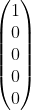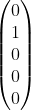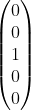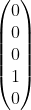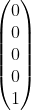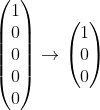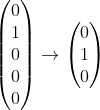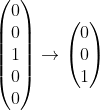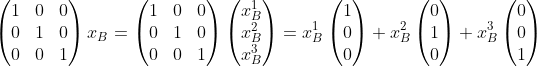说明基本可行解时说过，xB是在矩阵A中选择三个列作为单位矩阵得到的，同时提到，也可以选择矩阵其他的列作为3维空间的基，选择不同列作为3维空间基即可产生不同的xB，从而得到5维空间不同的顶点。新选择列称为入基，被替代的列称出基，这种替换的可以有许多种方式，使用暴力方法逐个迭代每一个顶点也可以实现，而当求解最小值问题时，如果借助梯度的概念，在得到一个顶点后，切换下一个顶点方向是函数变小的方向无疑会节省很多时间。

约束条件系数矩阵A中将作为基的列集合记作B，不是基的列集合记作N,则有A=(N，B),同样把x分量中基变量的集合记作xB，非基变量集合记作xN,约束条件可以写成： (N，B)（xNxB）=b,得到等式 NxN+BxB=b,矩阵B是基矩阵且可逆，得到:

xB=B-1b - B-1NxN                   ⑴

f(x)=cBxB+cNxN                ⑵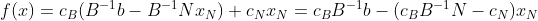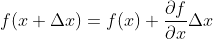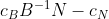越大则导数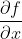越小,目标函数变小的幅度就越大，将此作为选择入基的标准，遍历每个非基列pj,由于每次都只一个列作为入基，xN其他分量依然为0，简化为cBB-1pj-cj,引入每一个待选列的检验量tj=cBB-1pj-cj, 如果检验量大于0，就选择这其中检验量最大的列作为入基就能保证目标函数变小，如果待选列的检验量都小于等于0则代表此时已经得到最小值。

入基已经准备就绪如何选择出基呢，即如何选择被替代的基列？在选择入基时其实还有一个隐含的问题，入基被选择后，新的基变量值是多少？这些可以从公式（1）中得到答案。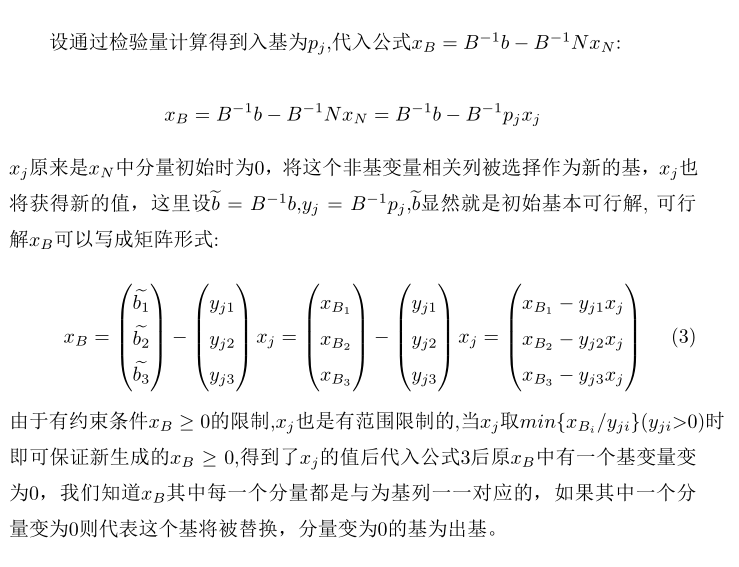```import numpy as np
BASEINDEX=2
#列变换实现单纯形法
def simplex_ColTranslate(c ,A,b,flagstable):
B=A[:,BASEINDEX:]
Binv=np.linalg.inv(B)
xb=np.dot(Binv  ,b)
cb=c[:,BASEINDEX:]
f=np.dot(cb,xb )
Inbaseindex=-1
OutBaseIndex = -1
tempvalue=0
for i in range(BASEINDEX):
v=np.squeeze(np.dot(np.dot(cb,Binv),A[:,i])-c[:,i],0)
if tempvalue<=v:
#找出入基
tempvalue=v
Inbaseindex=i
if tempvalue==0:
#找到最优解
return  xb,f,c,flagstable
else:
#入基出基替换，同时交换系数c
y=np.dot(Binv,A[:,Inbaseindex])
minivalue=None
for i in range(y.shape):
if y[i]>0:
x=xb[i]/y[ i]
#找出最小值，保证xb>=0
if minivalue is None or minivalue>x :
minivalue=x
OutBaseIndex=i+BASEINDEX
for i in range(A.shape):
if i==OutBaseIndex:
for j in range(A.shape):
tmp=A[j,i].copy()
A[j,i]=A[j, Inbaseindex]
A[j, Inbaseindex]=tmp
tempc= np.squeeze( c[:,OutBaseIndex],0).copy()
c[:,OutBaseIndex]=c[:,Inbaseindex]
c[:,Inbaseindex]=tempc
flagstable[OutBaseIndex-BASEINDEX]=Inbaseindex
return simplex_ColTranslate(c, A, b,flagstable)

def useColTranslate():
BASEINDEX = 2
c = np.array([[-4, -1, 0, 0, 0]],dtype=np.float)
A = np.array([[-1, 2, 1, 0, 0], [2, 3, 0, 1, 0], [1, -1, 0, 0, 1]],dtype=np.float)
b = np.array([, , ],dtype=np.float)
# 记录下标变动情况
flagstable = {}
for i in range(c.shape - BASEINDEX):
flagstable[i] = BASEINDEX + i
x, f, c, flags = simplex_ColTranslate(c, A, b, flagstable)
print('最优解:%.2f' % (f[0, 0]))
for j in range(x.shape):
print('x%d=%.1f' % (flags[j] + 1, x[j, 0]))

if __name__ == '__main__':
useColTranslate()```3.2 单纯形法的表格方法

上面代码中设置了一个常量BASEINDEX用以指示A矩阵中基矩阵的开始索引，此后通过检验量将入基替换出基，将新的列置换出去，同时更新目标函数中的c向量的顺序，将cB与cN中分量同时完成置换，c中分量与x中分量如影随行，上面算法本质上是通过矩阵列变换实现单纯形法，好处是容易理解，与数学推导过程完全一致，弊端是打乱了原来矩阵列的顺序，不易于二次利用其中的参数，如在接下来介绍的两阶段法中需要二次利用矩阵A，列变换的处理方式为后期处理带来很大的难度。

行变换的方法也称单纯形法表格法，行变换不是将入基与出基对调，而是通过消元法将入基变成单位向量，在这个消原过程中，将初始参数b向量放到A矩阵右侧一起参与消元法，加入b后的矩阵称为增广矩阵，表格法选择检验量以及找到最值的过程都是与列变换一致的。初等行变换相当于矩阵A左乘一个矩阵，初等列变换相当于矩阵A右乘一个矩阵，两种变换殊途同归，都是得到一个与xB维度一样的向量，即基本可行解的非零部分，接下来举一个使用表格法的例子：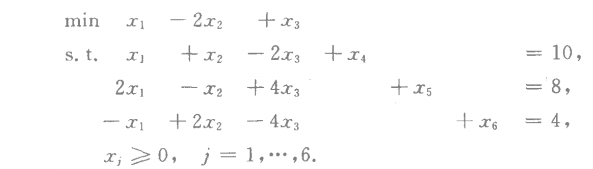接下来需要通过行变换把x2关联的非基向量(1,-1,2)变成单位向量(0,0,1)，方法是首先主元所在的第三行除以主元，将主元2变为1，然后将调整后第三行加上第二行使得-1变为0，再将第三行乘以-1加上第一行，这样x2关联的列向量(1,-1,2)就变成了(0,0,1)，而此时x6所关联的列不再是单位向量形式，代表已经变成了非基向量。注意这个行变换过程中，b也同时参与运算，这样单纯形表就变为下面的形式：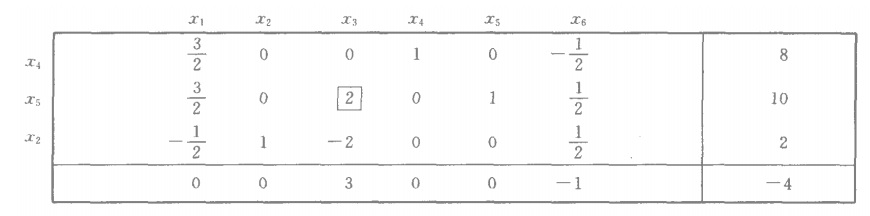```import numpy as np
#从矩阵得到单位基矩阵
def  getbaseIndexsfromMatrix(A):
index=[]
for i in range(A.shape):
if(np.dot(A[:,i],A[:,i].T)==1.0 and  np.max(A[:,i])==1.0 ):
index.append(i)
return  index
#从基变量表得到单位基矩阵
def getbaseIndexsfromVariables(flags):
index=[]
for key in flags:
index.append(flags[key])
return  index

#行变换-单纯形表格法,min=True求最小值
def simplex_RowTranslate(c ,A,b,flagstable,min=True):
#获得单位基矩阵
index=getbaseIndexsfromVariables(flagstable)
cb = c[:, index]
B=A[:,index]
Binv=np.linalg.inv(B)
tempvalue,Inbaseindex,OutBaseIndex  = 0,-1,-1
checkvalue=np.zeros(A.shape  )
for i in range(A.shape):
if i not in index:
v = np.squeeze(np.dot(np.dot(cb, Binv), A[:, i]) - c[:, i], 0)
checkvalue[i] = v
# 找出入基
if min:
if tempvalue < v:
tempvalue = v
Inbaseindex = i
else:
if tempvalue > v:
tempvalue = v
Inbaseindex = i
if tempvalue<=0 and min :
return b,   c, A,flagstable
elif tempvalue>=0 and min==False:
return b,   c, A, flagstable
else:
minivalue,privot,proitrow = None ,None,None
for r in flagstable:
if A[r,Inbaseindex] > 0:
x = b[r,0] /A[r,Inbaseindex]
if minivalue is None or minivalue>x :
minivalue=x
OutBaseIndex=flagstable[r]
#所在行，接下来要从该行开始做行变换
proitrow=r
#主元
privot=A[r,Inbaseindex]
A[proitrow,:]=A[proitrow,:]/privot
b[proitrow, 0] = b[proitrow, 0] / privot
for i in range(A.shape):
if i!=proitrow and A[i,Inbaseindex]!=0:
r = -A[i, Inbaseindex]
b[i, 0] = b[i, 0] + r * b[proitrow, 0]
for j in range(A.shape):
A[i, j]=A[i, j]+r*A[proitrow, j]
for key in flagstable:
if flagstable[key]==OutBaseIndex:
flagstable[key] = Inbaseindex
r2 = -checkvalue[Inbaseindex]
tempvalue=0
for i in range(checkvalue.__len__() ):
checkvalue[i] = r2 * A[proitrow, i] + checkvalue[i]
if min:
if tempvalue <= checkvalue[i]:
tempvalue = checkvalue[i]
else:
if tempvalue >= checkvalue[i]:
tempvalue = checkvalue[i]
if tempvalue<=0 and min:
return b,c, A, flagstable
elif tempvalue >= 0 and min == False:
return b,c, A, flagstable
else:
return simplex_RowTranslate(c, A, b, flagstable,min)

def useRowTranslate(  ):
c = np.array([[1, -2, 1, 0, 0,0]], dtype=np.float)
A = np.array([[1, 1, -2, 1, 0,0], [2, -1, 4, 0, 1,0], [-1, 2, -4, 0,0, 1]], dtype=np.float)
b = np.array([, , ], dtype=np.float)
# 基变量表
flagstable = {}
index = getbaseIndexsfromMatrix(A)
for i in range(index.__len__()):
flagstable[i] = index[i]

x, c, AA, flags = simplex_RowTranslate(c, A, b, flagstable)
index = getbaseIndexsfromVariables(flagstable)
cb = c[:, index]
B = AA[:, index]
Binv = np.linalg.inv(B)
f = np.dot(np.dot(cb, Binv), b)
print('最优解:%.2f' % (f[0,0] ))
for j in range(x.shape):
print('x%d=%.1f' % (flags[j] + 1, x[j, 0]))

if __name__ == '__main__':
useRowTranslate( )```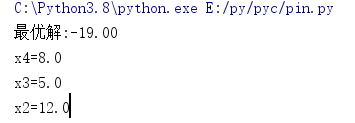4.1 人工变量的引入

上面的例子中在引入松弛变量变成标准型后，系数矩阵A自动有一个单位矩阵，单位矩阵的阶与条件个数一致，A秩为m的Rm*n的矩阵，即A是满秩的。有时加入松弛变量后并不能得到单位矩阵，遇到这种情形时自然不能得到初始基本可行解，比如下面这个例子: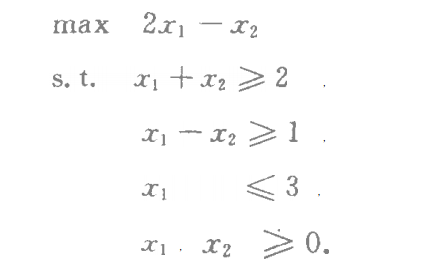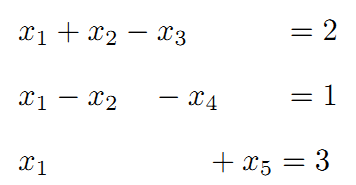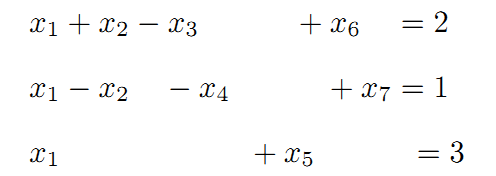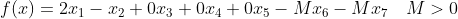-免费试读结束-

 上一篇  EM期望最大化算法 下一篇 一维搜索 评论区